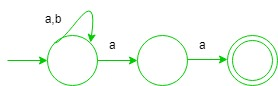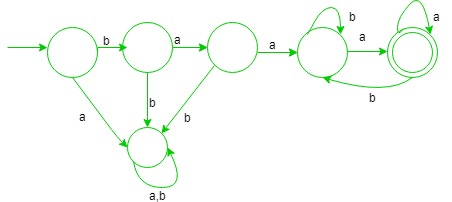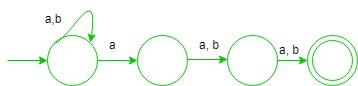# Automata Theory | Set 8

These questions for practice purpose for GATE CS Exam.

Ques-1: Which one of the following language is Regular?

(A) {wxwR | w,x ∈ (a+b)+}
(B) {wxwR | w ∈ (a+b)*, x ∈ {a,b}}
(C) {wwRx | w,x ∈ (a+b)+}
(D) {wwR | w ∈ (a+b)*}

Explanation:

• (A) It is correct, since this language can form regular expression which is {{ a(a + b)+a } + {b(a + b)+b}}, i.e., start and end with same symbol.
• (B) It is deterministic context free language since, string before and and after ‘x’ are same so, it is matched.
• (C) It cannot be regular since, wwR is done at first which requires comparison which cannot be done via finite automata.
• (D) It is also not regular since, comparison is required.

Option (A) is true.

Ques-2: Let w be any string of length n in {a, b}*. Consider ‘L’ be the set of all strings ending with at least n a’s. What is the minimum number of states in non deterministic finite automata that accept ‘L’?

(A) (n+3)
(B) (n+1)
(C) n
(D) 2n

Explanation:
It is correct since, the minimum number of states required for NFA for ending with at least 2 a’s is (2 + 1) i.e., regular expression will be (a + b)*aaHence, Number of states required for at least n a’s will be (n+1).

Option (B) is true.

Ques-3: What is the minimum number of states in deterministic finite automata (DFA) for string starting with ba2 and ending with ‘a’ over alphabet {a, b}?

(A) Ten
(B) Nine
(C) Eight
(D) Six

Explanation:In the above DFA, minimum number of states required is six.

Option (D) is correct.

Ques-4: Consider the following statements:

```S1 = {(an)m | n = 0}
S2 = {anbn | n>=1} U {anbm | n>=1, m>=1} ```

Which one of the following is regular?

(A) only S1
(B) only S2
(C) both S1 and S2
(D) none

Explanation:
Both given languages are regular. Option (C) is correct.

Ques-5: What is the number of states in minimal NFA(non deterministic finite automata), which accepts set of all strings in which the third last symbol is ‘a’ over alphabet {a, b}?

(A) three
(B) four
(C) six
(D) five

Explanation:In the above NFA, minimum number of states required is four.

Option (B) is true.My Personal Notes arrow_drop_upCheck out this Author's contributed articles.

If you like GeeksforGeeks and would like to contribute, you can also write an article using contribute.geeksforgeeks.org or mail your article to contribute@geeksforgeeks.org. See your article appearing on the GeeksforGeeks main page and help other Geeks.

Please Improve this article if you find anything incorrect by clicking on the "Improve Article" button below.

Article Tags :

1

Please write to us at contribute@geeksforgeeks.org to report any issue with the above content.